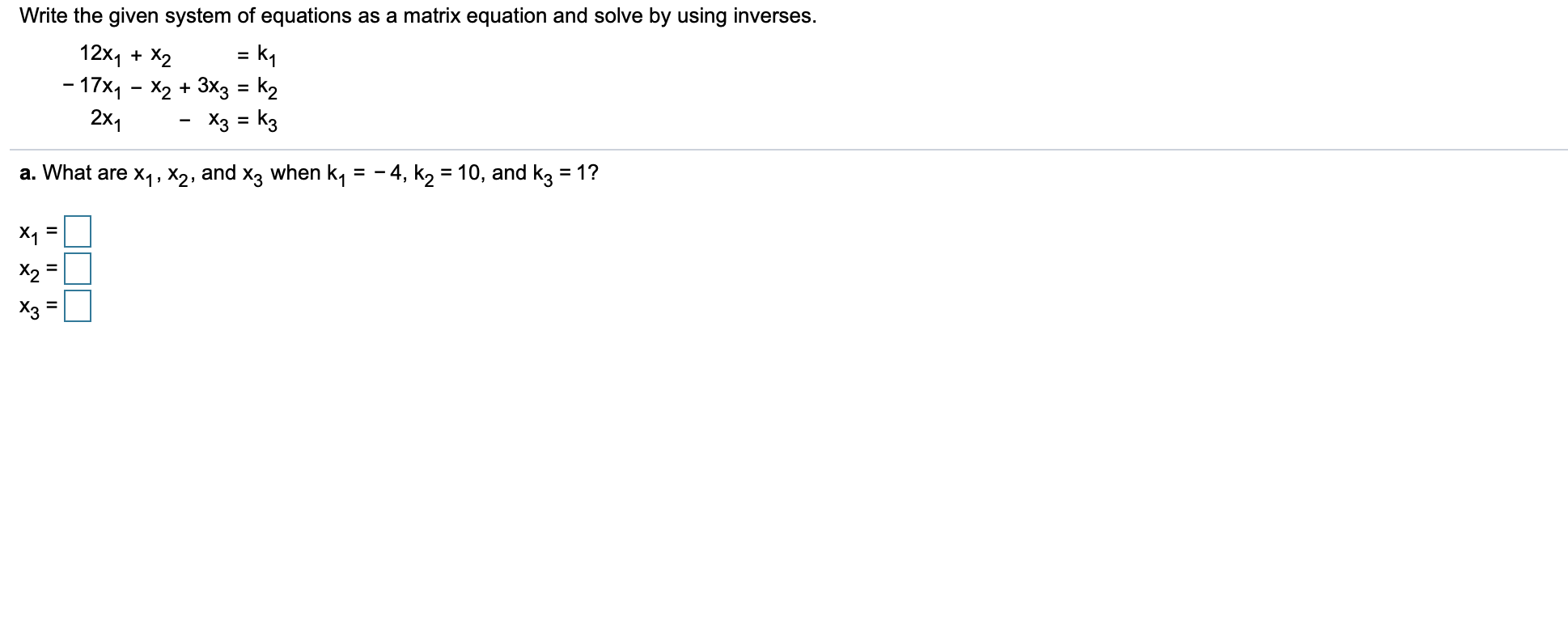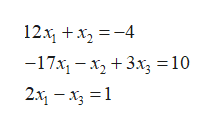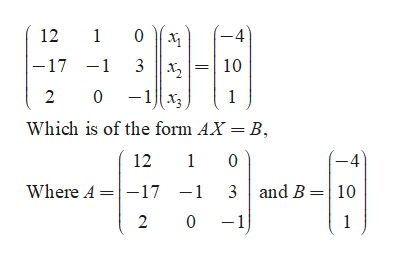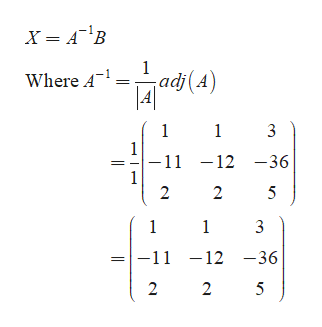# Write the given system of equations as a matrix equation and solve by using inverses.12x1X2-17x1 - X2 +3X3 = k2= k12x1Хз kзa. What are x1, X2, and x3 when k, = -4, k2 = 10, and k3 = 1?X1X2Хз

Question
1 viewshelp_outlineImage TranscriptioncloseWrite the given system of equations as a matrix equation and solve by using inverses. 12x1X2 -17x1 - X2 +3X3 = k2 = k1 2x1 Хз kз a. What are x1, X2, and x3 when k, = -4, k2 = 10, and k3 = 1? X1 X2 Хз fullscreen
check_circle

Step 1

Consider the system of linear equations,help_outlineImage Transcriptionclose12х, +х, —-4 -17х, — х, + 3х,%3D 10 2 м, — х, —1 fullscreen
Step 2

The matrix equation of the above linear system is,help_outlineImage Transcriptionclose12 1 0 -4 -17 -1 3 10 0 -1 x3 2 1 Which is of the form AX = B, 12 1 0 -4 and B 10 Where A 17 -1 3 2 01 1 fullscreen
Step 3

The variable X is calc...help_outlineImage Transcriptionclose1 adj (A) Where A 1 1 3 -11 -12-36 1 2 2 5 1 1 3 |-11 -12 -36 2 2 5 fullscreen

### Want to see the full answer?

See Solution

#### Want to see this answer and more?

Solutions are written by subject experts who are available 24/7. Questions are typically answered within 1 hour.*

See Solution
*Response times may vary by subject and question.
Tagged in

### Other